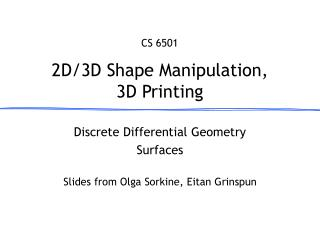DownloadDownload Presentation2D/3D Shape Manipulation, 3D Printing

# 2D/3D Shape Manipulation, 3D Printing

Télécharger la présentation## 2D/3D Shape Manipulation, 3D Printing

- - - - - - - - - - - - - - - - - - - - - - - - - - - E N D - - - - - - - - - - - - - - - - - - - - - - - - - - -
##### Presentation Transcript

1. CS 6501 2D/3D Shape Manipulation,3D Printing Discrete Differential Geometry Surfaces Slides from Olga Sorkine, EitanGrinspun

2. Surfaces, Parametric Form • Continuous surface • Tangent plane at point p(u,v) is spanned by n pv pu p(u,v) v u

3. Isoparametric Lines • Lines on the surface when keeping one parameter fixed v u

4. Surface Normals • Surface normal: • Assuming regular parameterization, i.e., n pv pu p(u,v) v u

5. Normal Curvature n pv pu p t Direction t in the tangent plane (if pu and pv are orthogonal): t Tangent plane 

6. Normal Curvature The curve  is the intersection of the surface with the plane through n and t. Normal curvature: n() = ((p)) n pv pu p t  t Tangent plane 

7. Surface Curvatures • Principal curvatures • Maximal curvature • Minimal curvature • Mean curvature • Gaussian curvature

8. Principal Directions • Principal directions:tangent vectorscorresponding to max and min t2 t1  max min curvature max curvature tangent plane

9. Principal Directions Euler’s Theorem: Planes of principal curvature are orthogonal and independent of parameterization.

10. Principal Directions

11. Mean Curvature • Intuition for mean curvature

12. Classification • A point p on the surface is called • Elliptic, if K > 0 • Parabolic, if K = 0 • Hyperbolic, if K < 0 • Umbilical, if • Developable surface iffK = 0

13. Isotropic:all directions are principal directions planar spherical (umbilical) K > 0 K = 0 K < 0 2< 0 2= 0 Anisotropic:2 distinct principal directions 2> 0, 1> 0 1> 0 1> 0 elliptic parabolic hyperbolic Local Surface Shape By Curvatures K > 0, 1= 2 K = 0

14. Gauss-Bonnet Theorem • For a closed surface M:

15. Gauss-Bonnet Theorem • For a closed surface M: • Compare with planar curves:

16. Fundamental Forms • First fundamental form • Second fundamental form • Together, they define a surface (given some compatibility conditions)

17. Fundamental Forms • I and II allow to measure • length, angles, area, curvature • arc element • area element

18. Intrinsic Geometry • Properties of the surface that only depend on the first fundamental form • length • angles • Gaussian curvature (TheoremaEgregium)

19. Laplace Operator gradientoperator 2nd partialderivatives Laplaceoperator Cartesiancoordinates function inEuclidean space divergenceoperator

20. Laplace-Beltrami Operator • Extension of Laplace to functions on manifolds gradientoperator Laplace-Beltrami function onsurface M divergenceoperator

21. Laplace-Beltrami Operator • For coordinate functions: gradientoperator mean curvature Laplace-Beltrami unitsurfacenormal function onsurface M divergenceoperator

22. Differential Geometry on Meshes • Assumption: meshes are piecewise linear approximations of smooth surfaces • Can try fitting a smooth surface locally (say, a polynomial) and find differential quantities analytically • But: it is often too slow for interactive setting and error prone

23. Discrete Differential Operators • Approach: approximate differential properties at point v as spatial average over local mesh neighborhood N(v) where typically • v = mesh vertex • Nk(v) = k-ring neighborhood

24. Discrete Laplace-Beltrami • Uniform discretization: L(v) or ∆v • Depends only on connectivity= simple and efficient • Bad approximation for irregular triangulations vi vj

25. Discrete Laplace-Beltrami • Intuition for uniform discretization

26. Discrete Laplace-Beltrami • Intuition for uniform discretization vi-1 vi vi+1 

27. Discrete Laplace-Beltrami • Intuition for uniform discretization vj1 vj2 vi vj6 vj3 vj5 vj4

28. Discrete Laplace-Beltrami • Cotangent formula vi ij vi Ai vi ij vj vj vj

29. Voronoi Vertex Area • Unfold the triangle flap onto the plane (without distortion) vi θ vj

30. Voronoi Vertex Area Flattened flap vi vi cj+1 θ cj vj

31. Discrete Laplace-Beltrami • Cotangent formula • Accounts for mesh geometry • Potentially negative/infinite weights

32. Discrete Laplace-Beltrami • Cotangent formula • Can be derived using linear Finite Elements • Nice property: gives zero for planar 1-rings!

33. Discrete Laplace-Beltrami vi • Uniform Laplacian Lu(vi) • Cotangent Laplacian Lc(vi) • Mean curvature normal b a vj

34. Discrete Laplace-Beltrami vi • Uniform Laplacian Lu(vi) • Cotangent Laplacian Lc(vi) • Mean curvaturenormal • FornearlyequaledgelengthsUniform≈Cotangent b a vj

35. Discrete Laplace-Beltrami vi • Uniform Laplacian Lu(vi) • Cotangent Laplacian Lc(vi) • Mean curvaturenormal • FornearlyequaledgelengthsUniform≈Cotangent b a vj CotanLaplacian allows computing discrete normal

36. Discrete Curvatures • Mean curvature (sign defined according to normal) • Gaussian curvature • Principal curvatures Ai j

37. Discrete Gauss-Bonnet Theorem • Total Gaussian curvature is fixed for a given topology

38. Example: Discrete Mean Curvature

39. Links and Literature • M. Meyer, M. Desbrun, P. Schroeder, A. BarrDiscrete Differential-Geometry Operators for Triangulated 2-Manifolds, VisMath, 2002

40. Links and Literature • P. Alliez, Estimating Curvature Tensors on Triangle Meshes, Source Code • http://www-sop.inria.fr/geometrica/team/Pierre.Alliez/demos/curvature/ principal directions

41. Measuring Surface Smoothness

42. Links and Literature • Grinspun et al.:Computingdiscrete shape operators on general meshes, Eurographics 2006

43. Reflection Lines as an Inspection Tool • Shape optimization using reflection linesE. Tosun, Y. I. Gingold, J. Reisman, D. ZorinSymposium on Geometry Processing 2007

44. Reflection Lines as an Inspection Tool • Shape optimization using reflection linesE. Tosun, Y. I. Gingold, J. Reisman, D. ZorinSymposium on Geometry Processing 2007

45. Thank You